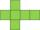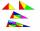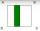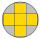# Cube wall

The perimeter of one cube wall is 120 meters. Calculate the surface area and the body diagonal of this cube.

Result

S =  5400 m2
u =  51.962 m

#### Solution:Leave us a comment of example and its solution (i.e. if it is still somewhat unclear...):

Showing 0 comments:Be the first to comment!#### To solve this example are needed these knowledge from mathematics:

Pythagorean theorem is the base for the right triangle calculator.

## Next similar examples:

1. Wall diagonalCalculate the length of wall diagonal of the cube whose surface is 384 cm square.
2. Grid/network of cubeGrid of cube has a circumference 42 mm. Calculate the surface area of the cube ...
3. Body diagonalCalculate the length of the body diagonal of the 6cm cube.
4. Body diagonalFind the cube surface if its body diagonal has a size of 6 cm.
5. Pyramid four sidesIn a regular tetrahedral pyramid is a body height 38 cm and a wall height 42 cm. Calculate the surface area of the pyramid; the result round to square centimeters.
6. LandRectangular triangular land has area 30 square meters and 12 meters long leg. How many meters of the fence do you need for fencing this land?
7. Isosceles triangleCalculate area and perimeter of an isosceles triangle ABC with base AB if a = 6 cm, c = 7 cm.
8. RT 11Calculate the area of right tirangle if its perimeter is p = 45 m and one cathethus is 20 m long.
9. RT 10Area of right triangle is 84 cm2 and one of its cathethus is a=10 cm. Calculate perimeter of the triangle ABC.
10. ISO trapezoid v2bases of Isosceles trapezoid measured 12 cm and 5 cm and its perimeter is 21 cm. What is the are of a trapezoid?
11. Folded squareABCD is a square. The square is folded on the midpoint of AB and A is folded onto the fold, creating a shaded region. The perimiter of the shaded figure is 75. Find the area of square ABCD
12. Equilateral triangleThe equilateral triangle has a 23 cm long side. Calculate its content area.
13. Square diagonalCalculate the length of diagonal of the square with side a = 23 cm.
14. ChordIt is given to a circle k(r=6 cm) and the points A, B such that / AB / = 8 cm lies on k. Calculate the distance of the center of circle S to the midpoint C of the segment AB.
15. Rectangular triangle PQRIn the rectangular triangle PQR, the PQ leg is divided by the X point into two segments of which longer is 25cm long. The second leg PR has a length 16 cm. The length of the RX is 20 cm. Calculate the length p of side RQ. The result is round to 2 decimal
16. Silver medalTo circular silver medal with a diameter of 10 cm is inscribed gold cross, which consists of five equal squares. What is the content area of silver part?
17. Holidays - on poolChildren's tickets to the swimming pool stands x € for an adult is € 2 more expensive. There was m children in the swimming pool and adults three times less. How many euros make treasurer for pool entry?